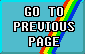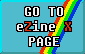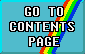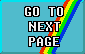# FIRST STEPS IN MACHINE CODE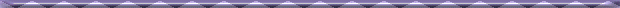## PART 2: WORKING WITH REGISTERS#### An introduction to Z80 Machine Code by David Nowotnik.

Last time I introduced to you the concepts of the Z80 micro-processor, machine code and assembly language programming. Using those concepts, I gave a few simple examples of moving data to and from the Z80 CPU. To remind you of the internal layout of this CPU, the diagram of the CPU is shown again this time (Figure 1) as I'll be referring to it again as I develop some more of the ways in which machine code can transfer data from memory to the internal registers of the CPU. But before we begin with these aspects of the machine code, I'd like to introduce you to a different way to deal with numbers when working with computers. This numeric system is called hexadecimal (commonly abbreviated to hex).The numeric system we use in every day life is called decimal. To use decimal, we have 10 characters (0 to 9) which we combine to define a number. Decimal is easy for us to learn as we have ten fingers to use in counting.

The computer, however, finds decimal cumbersome as it has eight 'fingers'; the number of bits in a register or bytes in memory. When using BASIC, the interpreter in ROM translates numbers to decimal to make life easier for us. But in using machine code, it becomes easier to adopt the computer's numeric system. This is a system which has 16 characters ('two hands' of eight 'fingers') which make up all numbers, and is called hexadecimal.

Hexadecimal works like this: The numbers 0 to 9 in decimal are the same in hexadecimal (or hex, for short). 10 decimal becomes 'A' in hex, eleven is 'B', and so on up to 15, which is 'F' hex. Sixteen is then 'l0' hex, and 26 decimal is '1A'; hex. The highest value in hex with just two characters is 'FF', which is 255 in decimal. And that just happens to be the highest value that can be stored in a byte (or in a single register). So, every byte value can be defined by a two character number using hex (e.g. 0A for ten, FA for 254 decimal).

Some of the other advantages of hex will become apparent as we go through the series. To encourage you to use hex rather than decimal, I have incorporated a hex/decimal conversion program, and, later, two new machine code loaders which use hex rather than decimal, as was used last time. But, for now, back to machine code.I introduced last time the assembly language command of LOAD. It happens to be the most frequently used machine code operation of all. There are several variations on LOAD; the simpler and most frequently used will now be described.

The simplest of all are the series of LOAD commands which allow the contents of one register to be copied into another register. The BASIC equivalent is LET B = C (for LD B, C); the originating register remains unaltered, but the value it holds (a number between 00 and FF hex) is copied into the other register. A single opcode carries out this operation; table 1 displays the opcodes for all possible inter-register transfers. There is no equivalent instruction to copy the contents of one register pair into another pair, but this can be achieved by two single register transfers, e.g.

LD H, B
LD L, C

to copy BC into HL.

It is, of course, necessary to load values into the registers from outside the CPU. One way to do this is to load data from the program. For example, the instruction:

LD B, n

will load the B register with a value represented by 'n'. In machine code, LD B, n appears as a two byte instruction. The first byte is 06 hex, which is the opcode for LD B, n. The value in the memory address immediately following this opcode is the value to be loaded into the B register. This is known as the operand. For example, if the two bytes (opcode and operand) were 06FB, then the value FB would be loaded into the B register. All the other registers can be loaded from the program in the same way, and all the opcodes for these instructions appear in table 2. Remember, you must have a second byte to complete this instruction.

You can, if you wish, load a register pair directly with a single instruction, rather than use two instructions. From last time, you will recall that the H and L, B and C, and D and E registers can be paired such that they effectively can hold any number between 0 and 65535 (00 to FFFF hex). C, E, and L form the low byte of the pair, while B, D, and H are the high bytes.

Three bytes make up the machine code instruction to load a register pair directly. The first is the opcode, the second is the value which goes in the low byte, and the third the value to go in the high byte. Note that the low byte precedes the high byte in a two byte number. This is a Z80 conversion, and we'll see more of that in a moment. The two byte load instructions also appear in Table 2.

The direct load instructions are equivalent to, for example, LET B = 5, or LET BC = 1225 in BASIC. For more flexibility, you may wish to load the equivalent of a variable (i.e. the contents of an address in memory) into one of the registers. There are a number of ways you can do this.

For reasons which will become apparent later, the A register is special. For example, it is the register in which all one byte arithmetic is carried out. So, there are more options to LOAD A than any other single register. You can load the A register with the contents of a byte from a specific memory address with LD A, (nn). 'nn' represents a memory address, and you may remember from last time that the brackets mean 'the contents of'. LD A, (nn) is a 3 byte instruction in machine, code; the fist byte (3A) is the opcode, the second and third are the low and high byte respectively of the address of the byte whose value is loaded into A.

Similarly a register pair can be loaded directly from memory; i.e. LD dd, (nn), where dd represents HL, DE, or BC. When dd is HL, there is a single opcode (2A), followed by a two byte operand. To load BC or DE a two byte opcode is required, followed by the address operand. The address operand points to the byte whose value is loaded into the low register of the pair; the next address in memory after the operand address is loaded into the high register of the pair.

For yet more flexibility, you can use the HL register pair to point to an address in memory whose value is loaded into a register. Thus, LD r, (HL) - where r represents any register - is an instruction requiring only one byte (the opcode) which takes the number stored in the HL register as the address in memory from which a value is copied into the register. The A register allows the BC and DE register pairs to perform as pointers as well (e.g. LD A, (BC)).

Whenever it is possible to load a register (or register pair) from an address in memory, it is also possible to copy the register value into memory. For example, LD (HL), A will copy the contents of the A register to an address in memory indicated by the value of the HL register.

The final LOAD instruction for now allows you to place a value into RAM without going through one of the registers of the CPU. That is LD (HL), n. A two byte instruction, the second being the value which goes into the address indicated by the value held in the HL register pair.

Well, that was a lot of theory in a short space. If it wasn't clear, read the section again, and look at Tables 2 and 3, which contain opcodes for all the LOAD instructions dealt with just now. Several of them will appear in the examples I give later on, which should also help to clarify these instructions.#### SIMPLE ARITHMETIC

Computers wouldn't get very far if all you could do was to transfer bytes of data from one place to another. Much of the rest of the series will deal with how registers can be manipulated; we'll start now with some simple arithmetic. Next issue I'll demonstrate addition and subtraction in machine code, but if all you want to do is add or subtract the number one from a register (or register pair) then the Z80 provides a simple way of doing it.

The instructions INC and DEC will, respectively, add or subtract one from any register or register pair. All these instructions are just one byte long (the opcode), and their values are shown in table 4. As an example, if the value of the A register is 3A, then INC A will increase it to 3B, and DEC A will decrease it to 39 hex. If the value of A were FF (the highest possible) then INC A would convert the value in A to zero; similarly, DEC A when A is zero would make A hold the value FF.

On paired registers, INC and DEC work on the combined value held by the pair of registers. So, beware, INC HL is not the same as INC H and INC L.

Again, the examples should clarify any doubts about INC and DEC.#### A SIMPLE LOOP

With the machine code I have given you so far, it would only be possible to go to the start of a code (the USR function), carry out a list of instructions, and, with the RET instruction, return to BASIC. From BASIC, you will have learnt the power and utility of FOR... NEXT loops; it's possible to do a similar thing in machine code. The simplest way is with a complex instruction DJNZ n.

The letters stand for Decrement B and Jump if Not Zero. What it does is to use the B register as a counter, just like the variable in a FOR... NEXT loop. When this instruction is encountered, the B register is decremented, and its value is tested. If it is not equal to zero, then the Program Counter (remember that from last time?) will jump to a value governed by the value of n, the operand to DJNZ (this is a 2 byte instruction). If the value of B is zero, then PC is incremented in the normal way, such that the next instruction (immediately following the DJNZ instruction) is implemented.

How the value of n controls the jump is quite complicated. It allows you to jump both forward and backwards; this is called relative jumping (as PC is altered relative to its current position). Here's how it works;

If the value of n is between 0 and 127, then the program counter jumps forward by the value of n; i.e. PC = PC + n. But if the value of n lies between 128 and 255, then the program counter jumps back according to the sum PC = PC - (256 - n). The most commonly occurring fault in writing machine code is the miscalculation of a relative jump, and there is little wonder why! One of the great benefits of writing in assembly language, and having an assembler program translate to machine code is that the calculation is carried out for you. If you do calculate relative jumps for yourself, then remember that the starting point for the sum is the address of the opcode immediately following the DJNZ instruction. That's the place you would end up if you had DJNZ 0.

For simple loops in machine code, you will be jumping back following the DJNZ instruction, and you'll see that in the examples I will be giving. You'll also notice that every time a loop with DJNZ is set up, the B register is filled with an appropriate value, much like the FOR statement in a FOR... NEXT loop.

One final opcode for now; you'll see it in some of the examples - that is NOP. It simply means do nothing! It is machine codes way of carrying out PAUSE. NOP only slows down machine code for a fraction of a second, so you'll need quite a few NOP's (in a loop) to see any effect.

Phew! That was a lot of theory in a small space. Let's try some examples now as light relief, and hopefully, to clarify the theory.#### EXAMPLES

First enter my machine code hex loader, then save it. The machine code appears in DATA statements in line 1000 onwards, so for each example, type in the DATA lines appropriate for that example. In all cases leave line 2000 as the last line; this contains a symbol ('s') that informs the program you have come to the end.

From the details given in the assembly language listing you should be able to work out what is happening. All examples use the Spectrum's display or attribute file, so the effect is always visual.

With the appropriate DATA lines in place (RUN the program, then call the machine code with RANDOMIZE USR 30000, and see what happens. Good luck!

##### VIEW EXAMPLE ASSEMBLY LANGUAGE LISTINGS#### OPCODES